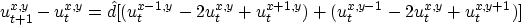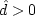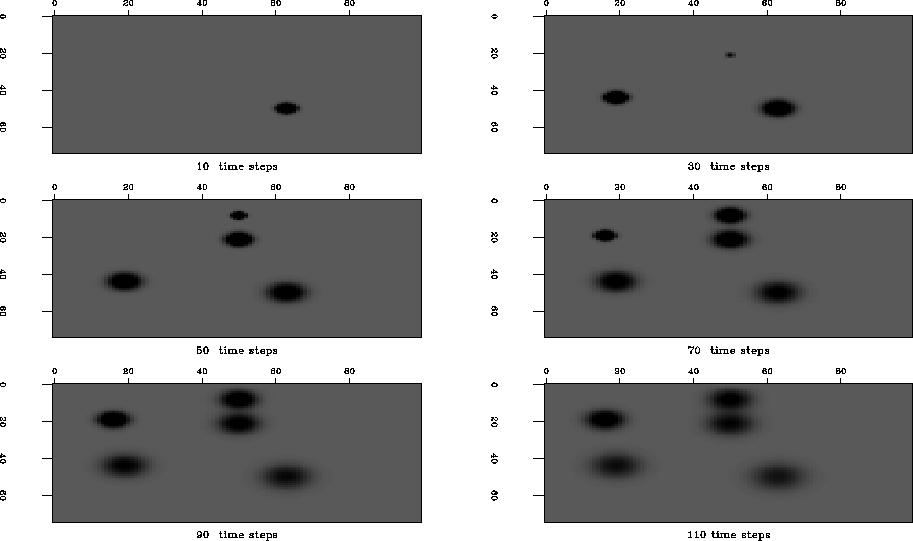Next: A first reservoir flow Up: Reservoir fluid flow simulator Previous: Reservoir fluid flow simulator

## Implementation of a reservoir flow simulator

The finite difference reservoir flow simulator for a single phase is a straightforward explicit finite difference implementation of the corresponding fluid flow equation 8:whereis the transmissibility. Each grid point represents a homogeneous subsurface cell. A straightforward stability analysis (see the finite difference wave equation section) yieldsand. Figure 1 shows snapshots of a reservoir of constant transmissibility and random sources of pressure. The pressure impulses diffuse over time as we would expect from a solution to a parabolic differential equation.impFlowSnap
Figure 1
Snapshots of the reservoir fluid flow simulator for a medium of constant transmissibility and randomly located point sources of pressure. The panels show the pressure field as time increases from left to right and top to bottom. An impulsive source generates an impulse that diffuses over time.Next: A first reservoir flow Up: Reservoir fluid flow simulator Previous: Reservoir fluid flow simulator
Stanford Exploration Project
3/9/1999